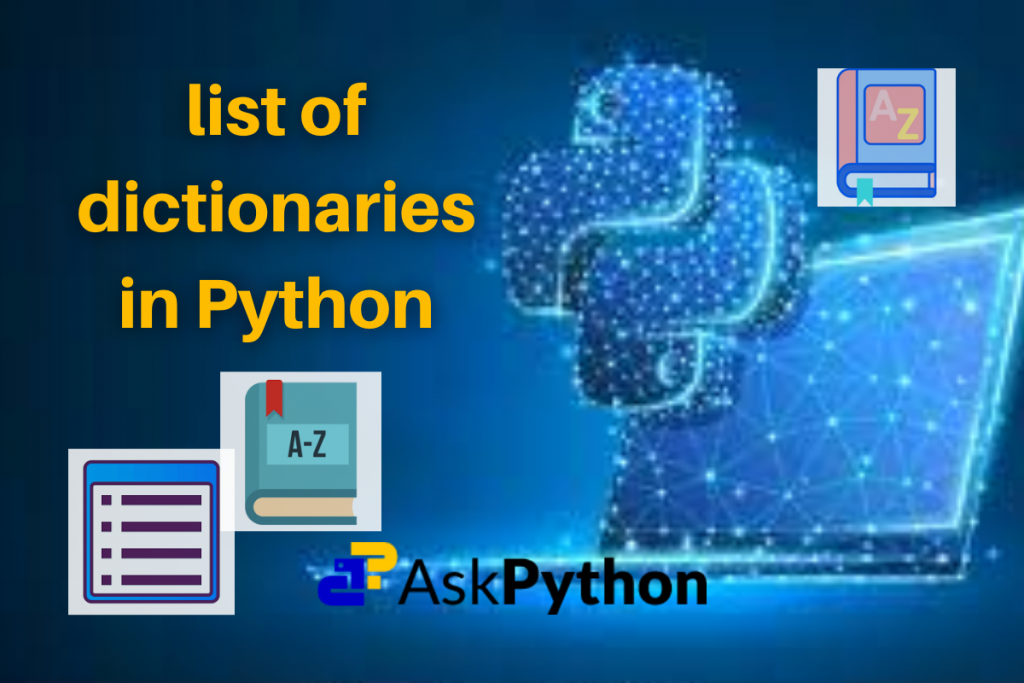# How to create a List of Dictionaries in Python?In this tutorial, we are going to discuss a list of dictionaries in Python. We will discuss how to

• create a list of dictionaries
• access the key:value pair
• update the key:value pair
• append a dictionary to the list

So without any further ado, let’s get started.

## What is a list of dictionaries in Python?

We all know in Python, a list is a linear data structure that can store a collection of values in an ordered manner. And these values can be any Python object.

A dictionary is also a Python object which stores the data in the key:value format.

Hence we can create a Python `list` whose each element is nothing but a Python `dictionary`. That’s why we call such a type of Python `list` by a special name – list of dictionaries.

## Steps to create a list of dictionaries in Python

To create a list of dictionaries in Python we just have to create a simple Python list and ensure that each of its elements is a Python dictionary. Let’s implement this in Python.

```# Defining a list of dictionaries in Python
ls_dict = [{'py': 'Python', 'mat': 'MATLAB', 'cs': 'Csharp'},
{'A': 65, 'B': 66, 'C': 67},
{'a': 97, 'b': 98, 'c': 99}]

# Printing the results
print(ls_dict)

# Validating the type of 'ls_dict' and its element
print(type(ls_dict))
print(type(ls_dict))
```

Output:

## Append a dictionary to the list of dictionaries

We can also append a list of dictionaries with a new Python dictionary object as its element. We use the Python list `append()` method here.

This is similar to appending a normal Python list.

The only difference is that the argument which is passed to the `append()` method must be a Python dictionary. The `append()` method adds the passed Python object (here it’s a dictionary) to the end of the existing Python list of dictionaries.

For example, we will update the above-created list of dictionaries with a new dictionary object. Let’s write the Python code for that.

```# Defining a list of dictionaries in Python
ls_dict = [{'py': 'Python', 'mat': 'MATLAB', 'cs': 'Csharp'},
{'A': 65, 'B': 66, 'C': 67},
{'a': 97, 'b': 98, 'c': 99},]

# Printing the given list of dictionaries
print("Given Python list of dictionaries:\n", ls_dict)

# Creating a new Python dictionary
ds = {'AskPython': "Python", 'JournalDev': "ALL",
'LinuxforDevices': "Linux"}

# Appending the list of dictionaries with the above dictionary
# Using append() method
ls_dict.append(ds)

# Printing the appended list of dictionaries
print("Appended Python list of dictionaries:\n", ls_dict)
```

Output:

## Access the key:value pairs from the list of dictionaries

To access the key:value pair of a list of dictionaries first we have to access the dictionary using indexing. As we get the dictionary, we can easily access any key:value pair of the dictionary. Let’s understand this through Python code.

```# Defining a list of dictionaries in Python
ls_dict = [{'A': 65, 'B': 66, 'C': 67},
{'py': 'Python', 'mat': 'MATLAB', 'cs': 'Csharp'},
{'a': 97, 'b': 98, 'c': 99}]

# Printing the given list of dictionaries
print("Given Python list of dictionaries:\n", ls_dict)

# Accessing and printing the key: value pairs of a list of dictionary
print(ls_dict)
print(ls_dict['py'])
```

Output:

## Update the key:value pairs in the list of dictionaries

We can update the key: value pairs of any dictionary in the list of dictionaries in the following ways:

• Add a new key:value pair
• Update an existing key:value
• Delete an existing key:value pair

Let’s update the above Python list of dictionaries in all the above discussed ways through Python code.

```# Defining a list of dictionaries in Python
ls_dict = [{'A': 65, 'B': 66, 'C': 67},
{'py': 'Python', 'mat': 'MATLAB', 'cs': 'Csharp'},
{'a': 97, 'b': 98, 'c': 99}]

# Printing the given list of dictionaries
print("Given Python list of dictionaries:\n", ls_dict)

# Adding a new key: value pair to the 1st dictionary in the list
ls_dict['new_key'] = 'new_value'

# Updating an existing key: value pair in the 2nd dictionary in the list
ls_dict['py'] = 'PYTHON'

# Deleting an existing key: value pair from the 3rd dictionary in the list
del ls_dict['b']

# Printing the updated list of dictionaries
print("Updated Python list of dictionaries:\n", ls_dict)
```

Output:

## Summing-up

In this tutorial, we have learned what’s a list of dictionaries in Python, to create a Python list of dictionaries, to append a Python list of dictionaries, to access the different key: value pairs from a Python list of dictionaries using the list indexes and the dictionary keys, and to update a Python list of dictionaries in three different ways.